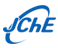SCI和EI收录∣中国化工学会会刊

• REACTION KINETICS, CATALYSIS AND…… •

### Kinetic Study of Atom Transfer Radical Polymerization of 2-（N,N-Dimethylamino） ethyl Methacrylate

JIANG Chengfa; ZHANG Yunxiang

1. Department of Chemical Engineering, Sichuan University, Chengdu 610065, China
• Received:1900-01-01 Revised:1900-01-01 Online:2004-04-28 Published:2004-04-28
• Contact: JIANG Chengfa

### 甲基丙烯酸-N, N-2-甲基乙胺酯原子转移自由基聚合动力学研究

1. Department of Chemical Engineering, Sichuan University, Chengdu 610065, China
• 通讯作者: 江成发

Abstract: A kinetic model was developed to describe the atom transfer radical polymerization (ATRP)
of 2-(N,N-dimethylamino) ethyl methacrylate (DMAEMA). The model was based on a
polymerization mechanism,which included the atom transfer equilibrium for primary radical,
the propagation of growing polymer radical, and the atom transfer equilibrium for the
growing polymer radical. An experiment was carried out to measure the conversion of
monomer, the number-average molecular weight of polymer and molecular weight distribution
for the ATRP process of DMAEMA. The experimental data were used to correlate the kinetic
model and rate constants were obtained. The rate constants of activation and deactivation
in the atom transfer equilibrium for primary radical are 1.0×10^4L·mol^-1·s^-1 and 0.04L
·mol^-1·s^-1, respectively. The rate constant of the propagation of growing polymer
radical is 8.50L·mol^-1·s^-1, and the rate constants of activation and deactivation in
the atom transfer equilibrium for growing polymer radical are 0.045L·mol^-1·s^-1 and 1.2
×10^5L·mol^-1·s^-1, respectively. The values of the rate constants represent the
features of the ATRP process. The kinetic model was used to calculate the ATRP process of
DMAEMA. The results show that the calculations agree well with the measurements.

of 2-(N,N-dimethylamino) ethyl methacrylate (DMAEMA). The model was based on a
polymerization mechanism,which included the atom transfer equilibrium for primary radical,
the propagation of growing polymer radical, and the atom transfer equilibrium for the
growing polymer radical. An experiment was carried out to measure the conversion of
monomer, the number-average molecular weight of polymer and molecular weight distribution
for the ATRP process of DMAEMA. The experimental data were used to correlate the kinetic
model and rate constants were obtained. The rate constants of activation and deactivation
in the atom transfer equilibrium for primary radical are 1.0×10^4L·mol^-1·s^-1 and 0.04L
·mol^-1·s^-1, respectively. The rate constant of the propagation of growing polymer
radical is 8.50L·mol^-1·s^-1, and the rate constants of activation and deactivation in
the atom transfer equilibrium for growing polymer radical are 0.045L·mol^-1·s^-1 and 1.2
×10^5L·mol^-1·s^-1, respectively. The values of the rate constants represent the
features of the ATRP process. The kinetic model was used to calculate the ATRP process of
DMAEMA. The results show that the calculations agree well with the measurements.